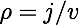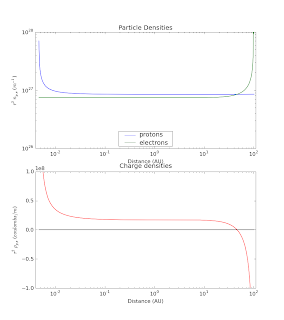## Thursday, December 18, 2008

### Electric Cosmos: The Solar Capacitor Model. II

Now we continue an examination of the Solar Capacitor model, concentrating on the particle fluxes implied by the model.

One of the most fundamental principles in this question is the continuity equation. We assume that electrons and protons make the complete trip between the solar photosphere and the heliopause. Any losses due to impacts with planets are small enough to be ignored in the total solar budget (though the impact on the planets themselves could be substantial) due to their small size. Therefore, it is simple to show that the flux of electrons, j_e, and of protons, j_p, at any radial position, r, other than the origin of the particles, can be found by:We also know that from the current density, j, and the mean particle speed, v, we can determine the charge density for electrons and protons by the fact thatFrom these relations, and the input parameters we've defined in the previous post, i.e.

Electron flux density at heliopause = 1e+9 electrons/m^2/s
Proton flux density at solar photosphere = 4.6e17 protons/m^2/s

Note that integrated over the area of their respective source surfaces, the total current will be the same for electrons and protons, a constraint that Dr. Scott claims will maintain charge neutrality.

From these boundary conditions and the physical relationships defined above, we can determine a number of characteristics of the particle fluxes at Earth orbit. They are summarized in the table below.

 Electrons Protons Speed (v/c): 0.999940 0.874387 Speed (m/s): 2.998e+08 2.621e+08 Energy (MeV): 4.606 995.394 Density (m^-3): 3.331e+04 3.810e+04 Flux (m^-2 s^-1): 9.986e+12 9.986e+12 Flux (cm^-2 s^-1): 9.986e+08 9.986e+08

Again, note that the fluxes are equal because they were chosen to pass the same total current at their respective sources. But also note that because the two flows have different speeds at the Earth's orbit, the charge densities will be different, a consequence of the continuity relationship above. With a surplus of protons, there is an excess positive charge density at this location of +4.8e3/m^3. We can use the same techniques to plot the charge distribution from the solar surface to the heliopause. The results are plotted in the figures below. To avoid the dramatic changes in scale because the flow density increases with decreasing radius, instead we scale the charge density by the charge density times the square of the radial distance. This gives us a measure of the amount of charge in each concentric spherical shell around the Sun.Figure 1a (top) shows the charge density distribution of electrons and protons individually in the solar capacitor model. The density is multiplied by r^2 to better illustrate the relative density of the two charge carriers. Figure 1b(bottom) illustrates the net charge density in the solar capacitor model. Again, the value is multiplied by r^2 to better illustrate the relative contribution of positive and negative charges.

One of the first results that jumps out at you is that over a large range of the distance within the heliopause, there is a NET POSITIVE CHARGE from 0.01AU to over 10AU.This is due to the fact that the fixed potential between the two electrodes (the Sun and the heliopause) create electron and proton streams that travel at different speeds. This keeps the charge densities different all along the path and the net charge does not balance to zero!

To be fair, one could tune the current emitted at the photosphere so that it more completely balances the electron charge density over this range.However, this requires extreme fine tuning. Matching the current densities of the electrons and protons does not guarantee a net charge density of zero, in contradiction to Dr. Scott's claim.

Next, let's compare these predictions of the Solar Capacitor model with some actual observations.

Plenty of satellites patrol the region between the Earth and the Sun: SOHO, ACE, Wind, more recently, STEREO A and B. They measure solar wind speed, composition, magnetic field, even electron energies. The data are all public. You can find some of it in places like the online archives and virtual observatories

If I examine some recent ACE data (December 14, 2008), we get fluxes for protons with energies greater than 10MeV protons of about 2 protons/cm^2/s. At energies above 30MeV, the flux drops to about half this value.

The solar capacitor model requires a flux of about 1e9 protons/cm^2/s at energies of around 990 MeV, nearly a billion times larger than the measured flux at this energy!

Next, we look at the low-energy (velocity) protons, such as from Table 1 of "Space Weather: The Solar Perspective" by Rainer Schwenn
http://solarphysics.livingreviews.org/open?pubNo=lrsp-2006-2&page=articlesu1.html
Here, we see that the low energy flux is very large, but those don't help Scott's model.

For solar wind electrons, we can check "Kinetic Physics of the Solar Corona and Solar Wind" by Eckart Marsch:
http://solarphysics.livingreviews.org/open?pubNo=lrsp-2006-1&amp;page=articlesu5.html
which shows that the electron velocity distribution in the solar wind at 1AU is not towards the Sun, as required in the solar capacitor model, but largely isotropic (with some enhancement along the magnetic field direction).

We can also examine the 27-day history of the solar electron flux at geostationary orbit
http://sd-www.jhuapl.edu/UPOS/MEV/index.html
Here, we see that in situ measurements demonstrate there is no stream of electrons inbound towards the Sun, contrary to the predictions of the solar capacitor model!

Next, let's compare the particle flux predicted for the Solar capacitor to the flux of particles trapped in the Earth's radiation belts

What do we find? Protons with an energy greater than 10MeV have a flux of 1e5/cm^2/s. Electrons with energies greater than 1MeV have a flux of 1e6/cm^2/s

These peak fluxes at these energies are fatal doses for astronauts! They also do a notorious amount of damage to spacecraft electronics unless the electronics are radiation hardened.

Yet the steady electron and proton fluxes of the solar wind predicted to power the solar capacitor model is 100-1000 times higher than the flux in the Earth's radiation belts!

Note that this is the STEADY flux in the solar capacitor model. What does it imply about the radiation shielding required to protect astronauts for interplanetary travel? What does it say for the prospects of humans going to other planets? Remember also, that the Moon is outside the Earth's magnetosphere, exposed to this flow, for most of its orbit. What chances do astronauts have on the Moon?

There are a number of interesting predictions by this model with the EU advocates don't talk about. Here's a couple off the top of my head.
1. What is the total energy in the outbound proton flow?
2. If you examine these results closely, you may find that they reveal another physical inconsistency in Scott's model. Can you identify it? Can you setup how you would solve it and show the results?

Can you think of more?